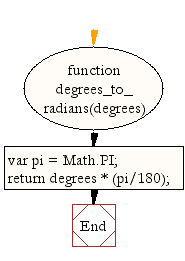# JavaScript: Convert an angle from degrees to radians

## JavaScript Math: Exercise-33 with Solution

Write a JavaScript function to convert an angle from degrees to radians.

Test Data :
0.7853981633974483

Use Math.PI and the degree to radian formula to convert the angle from degrees to radians.

Sample Solution-1:

JavaScript Code:

``````function degrees_to_radians(degrees)
{
var pi = Math.PI;
return degrees * (pi/180);
}

```
```

Sample Output:

```0.7853981633974483
```

Pictorial Presentation:Flowchart:Sample Solution-2:

JavaScript Code:

``````const degrees_to_radians = deg => (deg * Math.PI) / 180.0;
```
```

Sample Output:

```0.7853981633974483
1.5707963267948966
```

Live Demo:

See the Pen javascript-math-exercise-33 by w3resource (@w3resource) on CodePen.

Improve this sample solution and post your code through Disqus

What is the difficulty level of this exercise?

Test your Programming skills with w3resource's quiz.

﻿